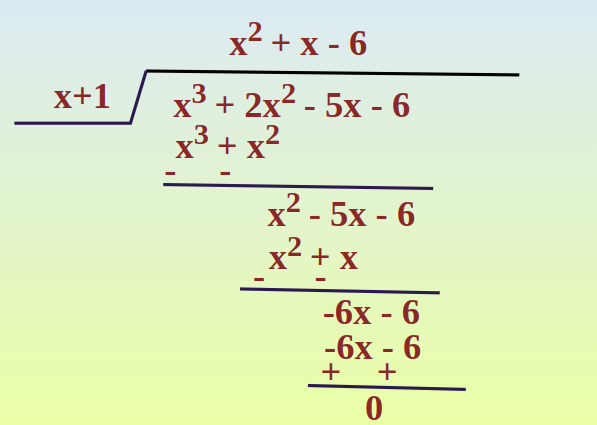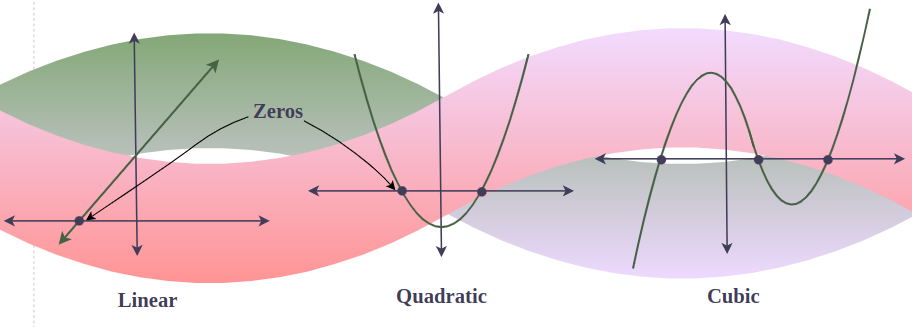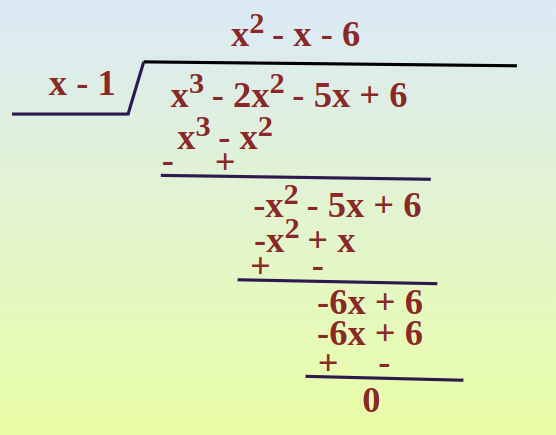Related Articles

# Zeros of Polynomial

Zeros of a Polynomial are those real, imaginary, or complex value when put in the polynomial instead of a variable, the result becomes zero (as the name suggest zero as well). Polynomials are used to model some physical phenomena happening in real life, they are very useful in describing situations mathematically. They are used in almost every field of Science, even outside of science for example in Economics and other related areas. Zeros or roots of these polynomials are a very important aspect of their nature and can be very useful while describing them or plotting them on a graph. Let’s look at their definition and methods of finding out the roots in detail.

## Zeros of Polynomials Definition

For a polynomial P(x), we say that x = a is the zero of the polynomial if P(a) = 0, and all such zeros of a polynomial are commonly called zeros of a polynomial. For example, consider f(x) = 3x – 12. Now, put x = 4 in the polynomial, i.e., f(4) = 3×4 – 12 = 0. Thus, x = 4 is a zero of polynomial f(x) = 3x – 12.

Example: For f(x) = x3 – 6x2 + 11x – 6, is x = 1 zero?

Solution:

To check whether if x = 1 is zero of f(x) = x3 – 6x2 + 11x – 6 or not, put x = 1 in (x)

f(1) = (1)3 – 6×(1)2 + 11×(1) – 6

⇒ f(1) = 1 – 6 + 11 – 6 = 12 -12 = 0

Thus, x = 1 is a zero of f(x).

## Zeros of Polynomial Formula

For a linear polynomial of form ax+b, its zero is given by x = -b/a.

For a quadratic polynomial of form ax2+bx+c, its zero is given by x = {-b±√D}/2a where D is Discriminant given by b2-4ac.

## How to Find Zero of a Polynomial?

We can find the zeros of the polynomial for various types of polynomials using various methods that are discussed below.

• For Linear Polynomial
• For Cubic Polynomial

### For Linear Polynomial

For Linear Polynomials, finding zero is the easiest of all. as there is only one zero and that can also be calculated by simple rearrangement of the polynomial after the equating polynomial to 0.

For example, find zero for linear polynomial f(x) = 2x – 7.

Solution:

To find zero of f(x), equate f(x) to 0.

⇒ 2x – 7 = 0

⇒ 2x = 7

⇒ x = 7/2

There are various methods to find roots or zeros of a quadratic polynomial such as splitting the middle term, a quadratic formula which is also known as the Shree Dharacharya formula, and completing the square which is somewhat similar to the quadratic formula, as quadratic formula comes from the completing the square for the general quadratic equation.

Example 1: Find out the zeros for P(x) = x2 + 2x – 15.

x2 + 2x – 15 = 0

⇒ x2 + 5x – 3x – 15 = 0

⇒ x(x + 5) –  3(x + 5) = 0

⇒ (x – 3) (x + 5) = 0

⇒ x = 3, -5

Example 2: Find the out zeros for P(x) = x2 – 16x + 64.

x2 – 16x + 64 = 0

Comparing with  ax2 + bx + c = 0,

we get, a = 1, b = -16, and c = 64.

Thus,⇒  x = 8, 8

### For Cubic Polynomial

To find zeros of cubic there are many ways, such as rational root theorem and long division together. One method of finding roots of cubic or any higher degree polynomial is as follows:

Step 1: Use the rational root theorem to find the possible roots. i.e., If a polynomial has a rational root it must be the division of p/q, where p is the integer constant and q is the leading coefficient.

Step 2: After finding one root, divide the polynomial with the factor formed by that root using long division and write the polynomial as a product of quotient and dividend.

Step 3: If the quotient is a quadratic expression solve it by the methods above mentioned for quadratic polynomials. If not a polynomial of a degree  2 then repeat steps 1 and 2 until the quotient becomes a polynomial with degree 2.

Step 4: The result of step 3 is the required factors, and by equating the factor to 0, we can find the zeros of the polynomial.

Example: Find the zeros of the cubic polynomial p(x) = x3 + 2x2 – 5x – 6.

Solution:

p(x) = x3 + 2x2 – 5x – 6

As p/q = -6

By rational root theorem, all possible rational roots of the polunomial are divisors of p/q.

Thus, divisors = ±1, ±2, ±3, ±6

x = -1, in p(x), we get

p(-1) = (-1)3 + 2(-1)2 – 5(-1) – 6

p(-1) = -1 + 2 + 5 – 6 = 0

Thus, by factor theorem, x + 1 is the factor of p(x).Thus, x3 + 2x2 – 5x – 6 = (x+1)(x2 +x – 6)

x3 + 2x2 – 5x – 6 = (x+1)(x-2)(x+3)

For zeroes, p(x) = 0,

Zeros of p(x) are x = -1, x = 2, and  x = -3.

## Factor Theorem

For the polynomial P(x), the factor theorem states that if x =a is zero of P(X) iff x – a is a factor of P(x). i.e., both the following conditions should hold true.

If a is a zero of P(x) then x−a will be a factor of P(x)
If x−a is a factor of P(x) then a will be a zero of P(x)

This can be verified by looking at previous examples. Factor theorem can lead to some interesting results, which are as follows:

Result 1: If P(x) is a polynomial of degree “n”, and “r” is a zero of P(x) then P(x) can be written in the following form,

P(x) = (x – r) Q(x)

Where Q(x) is a polynomial of degree “n-1” and can be found out by dividing P(x) with (x – r).

Result 2: If P(x) = (x-r)Q(x) and x = t is a zero of Q(x) then x = t will also be a zero of P(x).

To verify the above fact,

Let’s say “t” is zero Q(x), which means Q(t) = 0.

We know that “r” is a zero of polynomial P(x), where P(x) = (x – r) Q(x),

So we need to check if x = t is also a zero of P(x), let’s put x = t in P(x)

P(t) = (t – r) Q(t)  = 0

So, x = t is also a zero P(x).

Hence, Proved.

## Relation between Zeros and Coefficient

The relation between the zeros and the coefficient of the quadratic and cubic equation is discussed below.

### Relation between Zeros and Coefficient for Quadratic Equation

For a quadratic equation of the form ax2 + bx + c = 0, if the two zeros of the quadratic equation are α and β, then

• Sum of root = α + β = -b/a
• Product of roots = α × β = c/a

### Relation between Zeros and Coefficient for Cubic Equation

If α, β, and γ are the root of the cubic polynomial  ax3 + bx2 + cx + d = 0, then the relation between its zeros and coefficients is given as follows:

• α + β + γ = -b/a
• α × β × γ= -d/a
• αβ + αγ + βγ = c/a

## Forming Equation with Zeros of Polynomial

For a quadratic polynomial with zeros α and β, the quadratic polynomial is given by x2 – (α + β)x + αβ.

For a cubic polynomial with three zeros α, β, and γ, the cubic polynomial is given by x3 – (α + β + γ )x2 + (αβ + αγ + βγ)x – αβγ

## Zeros in Graph of Polynomials

In the graph of any polynomial y = f(x), real zeros are the point for which the graph intersects or touches the x-axis. (as a graph with an imaginary zero never cuts the x-axis). In other words, if there are 3 real solutions of a cubic polynomial then the graph of that cubic polynomial intersects the x-axis three times, but if there is only one real solution for some cubic polynomial then it graph only cuts the x-axis once.## Fundamental Theorem of Linear Algebra

If P(x) is a polynomial of degree “n” then P(x) will have exactly n zeros, some of which may repeat.

This means that if we list out all the zeroes and list each one k times when k is its multiplicity. We will have exactly n numbers in the list. This can be useful as it can give us an idea about how many zeros should be there in a polynomial. So we can stop looking for zeros once we reach our required number of zeros.

### Multiplicity of a Root

Suppose we have a polynomial P(x) = 0 which factorizes into,

P(x) = (x – r)k(x – a)m

If r is a zero of a polynomial and the exponent on its term that produced the root is k then we say that r has multiplicity k. Zeroes with a multiplicity of 1 are often called simple zeroes and zeros with a multiplicity of 2 is called double root of the polynomial.

Example: P(x) is a degree-5 polynomial, that has been factorized for you. List the roots and their multiplicity.

P(x) = 5x5−20x4+5x3+50x2−20x−40=5(x+1)2(x−2)3

Solution:

Given, P(x) = 5(x+1)2(x−2)

⇒ P(x) = 5(x+1)(x+1)(x+1)(x−2)(x−2)

To find zeros, P(x) = 0

⇒ x = -1, -1, 2, 2, 2

Notice that “-1” occurs two times as a zero, so its multiplicity is 2 while the multiplicity of the zero “2” is 3.

## Sample Problems on Zeros of Polynomial

Problem 1: Given that x = 2 is a zero of P(x) = x3+2x2−5x−6. Find the other two zeroes.

Solution:

From the fundamental theorem we studied earlier, we can say that P(x) will have 3 zeros because it is a three degree polynomial. One of them is x = 2.

So we can rewrite P(x),

P(x) = (x – 2) Q(x)

For finding the other two zeros, we need to find out the Q(x).

Q(x) can be found out by dividing P(x) by (x-2).

After dividing, the Q(x) comes out to be,

Q(x) = x2 + 4x + 3

The remaining two zeros can be found out from this,

Q(x) = x2 + 3x + x + 3

⇒ x(x + 3) + 1(x + 3)

⇒ (x + 1) (x + 3)

Q(x) = 0,

x = -1, -3

Thus, the other two zeros are x = -1 and x = -3.

Problem 2: Given that x = r is a zero of a polynomial, find out the other zeros of the polynomial.

P(x) = x3−6x2−16x; r = −2

Solution:

We know that x = -2 is a zero,

So, P(x) can be rewritten as, P(x) = (x + 2) Q(x) {By using Division Algorithm}

Now to find Q(x), we do the same thing as we did in the previous question, we divide P(x) with (x + 2).

We get,

Q(x) = x2 – 8x

Now to find the other two zeros, factorize Q(x)

Q(x) = x (x – 8) = 0

So, the zeros are x = 0, 8.

Thus, we have three zeros, x = -2, 0, 8.

Problem 3: Find the zeros of the polynomial, 4x3-3x2-25x-6 = 0

Solution:

Trick to solve polynomial equations with degree 3,

Find the smallest integer that can make the polynomial value 0, start with 1,-1,2, and so on…

we find that for x = -2 we get the value of expression to be zero.

Hence one of the roots is -2.

As per factor theorem if a is one of the zeros of the polynomial, hence (x-a) is factor of given polynomial.

Thus following this {x – (-2)} = (x+2) is a factor pof above polynomial.

(4x2-11x-3)(x+2) = 0

(4x2-12x+x-3)(x+2) = 0

[4x(x-3)+1(x-3)](x+2) = 0

(4x+1)(x-3)(x+2) = 0

x = -2, x = 3, x = -1/4

Problem 4: Find the zeros of the polynomial, 4x6– 16x4= 0

Solution:

The Polynomial has up to degree 6, hence, there exist 6 zeros of the polynomial.

4x4(x2-4) = 0

4x4(x2-22) = 0

4x4[(x+2)(x-2)] = 0

Therefore, x= 0, 0, 0, 0, 2, -2

Problem 5: Find the zeroes of the polynomial function f(x) = x3 – 2x2 – 5x + 6

Solution:

To find the zeros of this polynomial, we set f(x) = 0 and solve for x:

f(x) = x3 – 2x2 – 5x + 6 = 0

As d/a = 6

By rational root theorem, all possible rational roots of the polunomial are,

Divisors of d/a = ±1, ±2, ±3, ±6

x = 1, in p(x), we get

f(1) = (1)3 – 2(1)2 – 5(1) – 6

f(-1) = 1 – 2 – 5 + 6 = 0

Thus, by factor theorem, x – 1 is the factor of p(x).Thus, x3 + 2x2 – 5x – 6 = (x-1)(x2 -x – 6)

x3 + 2x2 – 5x – 6 = (x-1)(x+2)(x-3)

For zeroes, p(x) = 0,

Zeros of p(x) are x = 1, x = -2, and  x = 3.

## FAQs on Zeros of Polynomial

### Q1: What are Zeros of a Polynomial?

Those such real values, for the value of polynomial becomes 0 i.e., if p(x) is a polynomial, and p(a) = 0, then x = a is the zero of p(x).

### Q2: How to find the Zeros of a Polynomial?

There is various method for various different polynomials to find zeroes such as for quadratic spilling the middle term and quadratic formula. For linear, simple rearrangement of variables and for cubic we use a combination of rational root theorem, long division, factor theorem and remainder theorem.

### Q3: Can a Polynomial have more than One Zero?

Yes, a polynomial can have more than one zero, in fact, the polynomial of n degrees can have at most n real zeros.

### Q4: What is the Multiplicity of a Zero of a Polynomial?

In the factorization process, one factor or one zero of a polynomial then a number of times a factor or a zero came, that is called the multiplicity of that root.

### Q5: What is the Fundamental Theorem of Algebra?

The fundamental Theorem of algebra states “If P(x) is a polynomial of degree “n” then P(x) will have exactly n zeros, some of which may repeat.”

### Q6: Does a Polynomial with a Degree n always have n Real Roots?

No, a polynomial with degree n doesn’t always have n real roots, as some roots can be imaginary or complex numbers.